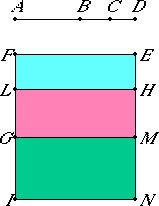# Proposition 81

To a second apotome of a medial straight line only one medial straight line can be annexed which is commensurable with the whole in square only and which contains with the whole a medial rectangle.
X.75

Let AB be a second apotome of a medial straight line and BC an annex to AB. Then AC and CB are medial straight lines commensurable in square only such that the rectangle AC by CB which they contain is medial.

I say that no other medial straight line can be annexed to AB which is commensurable with the whole in square only and which contains with the whole a medial rectangle.

X.75

If possible, let BD also be so annexed. Then AD and DB are also medial straight lines commensurable in square only such that the rectangle AD by DB which they contain is medial.II.7

Set out a rational straight line EF. Apply EG, equal to the sum of the squares on AC and CB, to EF producing EM as breadth. Subtract HG, equal to twice the rectangle AC by CB, producing HM as breadth. Then the remainder EL equals the square on AB, so that AB is the side of EL.

Again, apply EI, equal to the sum of the squares on AD and DB, to EF producing EN as breadth.

II.7

But EL also equals the square on AB, therefore the remainder HI equals twice the rectangle AD by DB.

Now, since AC and CB are medial straight lines, therefore the squares on AC and CB are also medial. And they equal EG, therefore EG is also medial.

X.22

And it is applied to the rational straight line EF, producing EM as breadth, therefore EM is rational and incommensurable in length with EF.

X.23,Cor.

Again, since the rectangle AC by CB is medial, twice the rectangle AC by CB is also medial. And it equals HG, therefore HG is also medial.

X.22

And it is applied to the rational straight line EF, producing HM as breadth, therefore HM is also rational and incommensurable in length with EF.

Since AC and CB are commensurable in square only, therefore AC is incommensurable in length with CB.

X.11

But AC is to CB as the square on AC is to the rectangle AC by CB, therefore the square on AC is incommensurable with the rectangle AC by CB.

But the sum of the squares on AC and CB is commensurable with the square on AC, while twice the rectangle AC by CB is commensurable with the rectangle AC by CB, therefore the sum of the squares on AC and CB is incommensurable with twice the rectangle AC by CB.

And EG equals the sum of the squares on AC and CB, while GH equals twice the rectangle AC by CB, therefore EG is incommensurable with HG.

But EG is to HG as EM is to HM, therefore EM is incommensurable in length with MH.

X.73

And both are rational, therefore EM and MH are rational straight lines commensurable in square only, therefore EH is an apotome, and HM an annex to it.

X.79

Similarly we can prove that HN is also an annex to it. Therefore to an apotome different straight lines are annexed which are commensurable with the wholes in square only, which is impossible.

Therefore, to a second apotome of a medial straight line only one medial straight line can be annexed which is commensurable with the whole in square only and which contains with the whole a medial rectangle.

Q.E.D.

## Guide

This proposition is not used in the rest of the Elements.